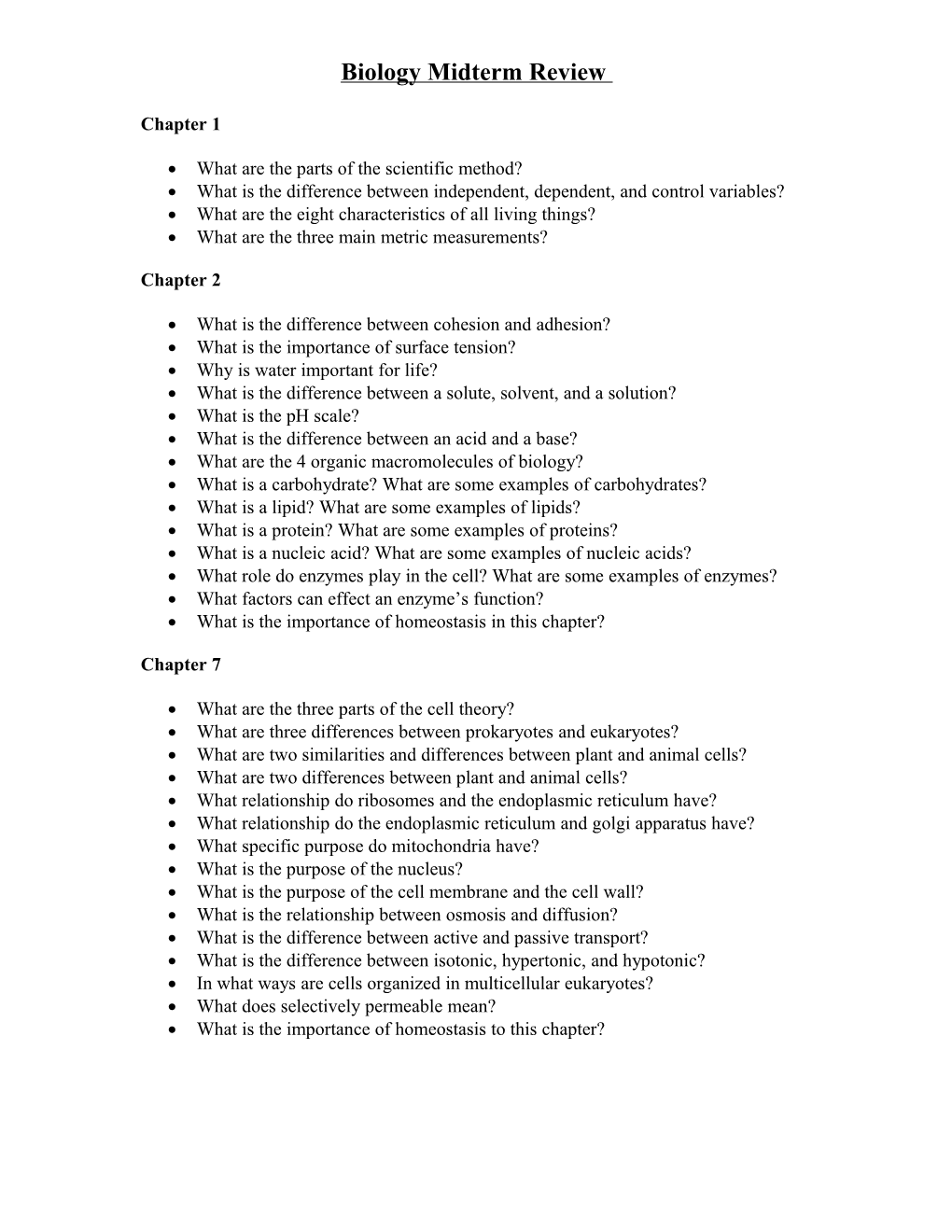# Biology Midterm ReviewBiology Midterm Review

Chapter 1

• What are the parts of the scientific method?
• What is the difference between independent, dependent, and control variables?
• What are the eight characteristics of all living things?
• What are the three main metric measurements?

Chapter 2

• What is the difference between cohesion and adhesion?
• What is the importance of surface tension?
• Why is water important for life?
• What is the difference between a solute, solvent, and a solution?
• What is the pH scale?
• What is the difference between an acid and a base?
• What are the 4 organic macromolecules of biology?
• What is a carbohydrate? What are some examples of carbohydrates?
• What is a lipid? What are some examples of lipids?
• What is a protein? What are some examples of proteins?
• What is a nucleic acid? What are some examples of nucleic acids?
• What role do enzymes play in the cell? What are some examples of enzymes?
• What factors can effect an enzyme’s function?
• What is the importance of homeostasis in this chapter?

Chapter 7

• What are the three parts of the cell theory?
• What are three differences between prokaryotes and eukaryotes?
• What are two similarities and differences between plant and animal cells?
• What are two differences between plant and animal cells?
• What relationship do ribosomes and the endoplasmic reticulum have?
• What relationship do the endoplasmic reticulum and golgi apparatus have?
• What specific purpose do mitochondria have?
• What is the purpose of the nucleus?
• What is the purpose of the cell membrane and the cell wall?
• What is the relationship between osmosis and diffusion?
• What is the difference between active and passive transport?
• What is the difference between isotonic, hypertonic, and hypotonic?
• In what ways are cells organized in multicellular eukaryotes?
• What does selectively permeable mean?
• What is the importance of homeostasis to this chapter?

Chapter 8 and 9

• What is ATP?
• What is the difference between autotrophs and heterotrophs?
• What major organelle is involved in photosynthesis? Cell respiration?
• What is necessary for photosynthesis? What are the products of photosynthesis?
• What is necessary for aerobic cell respiration? What are the products?
• What are the specific parts of photosynthesis? How are they different?
• What are the specific parts of aerobic cell respiration? How are they different?
• What is the difference between aerobic and anaerobic cell respiration?
• What is the difference between lactic acid and alcohol fermentation?
• What is the importance of homeostasis to these chapters?

Chapter 10

• What are the names of the 4 phases of mitosis?
• What happens in each phase of mitosis?
• What is cytokinesis?
• What are cancer cells?
• What happens during the cell cycle? Be specific about each phase.